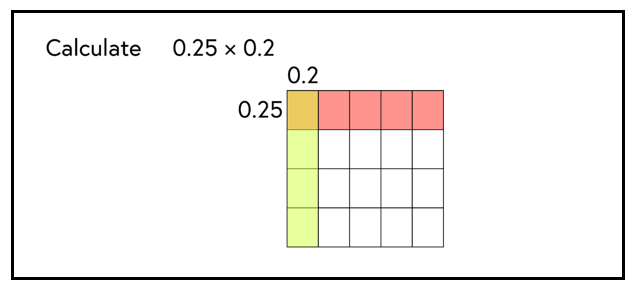3.2

## National STEM Learning Centre

Skip to 0 minutes and 7 secondsMICHAEL ANDERSON: So in this step, we're going to look at multiplying decimals when you don't have a calculator at hand. The example that we're going to look at is 0.25 multiplied by 0.2. Now, before getting stuck into this calculation, we're going to take a step back and consider the area of this square. So each side length is 1. And the area of the square is just 1 times 1. So it's 1 as well. Now, what we can do is, first of all, split the square into 4 equal parts. So each of the lengths now are 0.25.

Skip to 0 minutes and 41 secondsSo what we have as an area of each one of the rectangles is 0.25 multiplied by the length of 1, which gives us an area of each of them as 0.25. Now, we can do the same thing again. But this time, we're going to split from the top to the bottom. 0.2 is 1/5. So we're going to split this into 5 equal parts all the way down. And what we have there are a series of smaller rectangles, which is going to be represented by 0.25 and 0.2 to give them that area. So the area of each of these smaller rectangles is 0.25 multiplied by 0.2.

Skip to 1 minute and 24 secondsNow, when we look at the whole area, or the area as the whole, we have 20 of these smaller rectangles that make up the area of 1. Now, 1/20 of 1 gives us 0.05. so So rectangle is worth 0.05. And that's helped us to get to the answer of 0.25 multiplied by 0.2, giving 0.05.

# Multiplying decimals without using a calculator

When multiplying together small whole numbers such as $3 \times 6$ it is easy to visualise what this means. Students at a young age will recognise this as being three lots of six and will be able to show that this is the same as six lots of three.

At a young age students will use manipulatives, counters or blocks, to explain their working. However, attempting to explain what the calculation $0.62 \times 0.31$ means using counters or blocks is challenging, hence, how to multiply decimals can be approached by learning an algorithm with little underlying understanding.

In this step Michael demonstrates the use of a pictorial representation to help develop understanding of what is happening when we multiply 0.25 by 0.2.## Identify

Can you identify where this pictorial representation might not work?

How might we use a pictorial representation when the decimal is larger than 0.5, or larger than 1?